SAIL 新任负责人，Christopher Manning于1989年在澳大利亚国立大学取得三个学士学位（数学、计算机和语言学），并于 1994 年获得斯坦福大学语言学博士学位。 他曾先后在卡内基梅隆大学、悉尼大学等任教，1999 年回到母校斯坦福，就职于计算机科学和语言学系，是斯坦福自然语言处理组（Stanford NLP Group）的创始成员及负责人。重返斯坦福之后，他一待就是 19 年。 Manning 的研究目标是以智能的方式实现人类语言的处理、理解及生成，研究领域包括树形 RNN 、情感分析、基于神经网络的依存句法分析、神经机器翻译和深度语言理解等，是一位 NLP 领域的深度学习开拓者。他是国际计算机学会 (ACM)、国际人工智协会（AAAI）、国际计算语言学会（ACL）等国际权威学术组织的 Fellow，曾获 ACL、EMNLP、COLING、CHI 等国际顶会最佳论文奖，著有《统计自然语言处理基础》、《信息检索导论》等自然语言处理著名教材。0+0+0+0+0+0+0+0+0+0+0+0+0+0+0+0+2+0+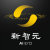0+1+0+0+0+0+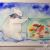0+0+0+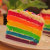0+1+0+0+0+0+0+2+0+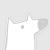0+0+0+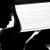0+0+0+0+5+0+
Top